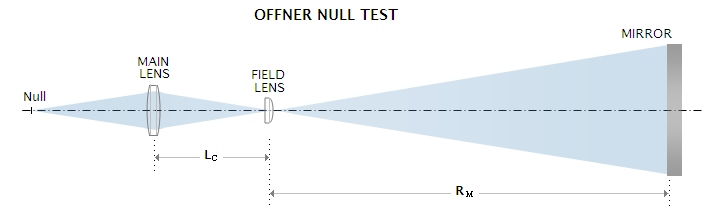telescopeѲptics.net          ▪▪▪▪                                             CONTENTS

# 4.8.7. Offner null test

Yet another alternative using lens to offset spherical aberration of a mirror at the center of curvature (where is placed light source) is Offner null test, which uses two axially positioned plano-lenses, facing the mirror with their curved surfaces - one with the front surface coinciding with the mirror center of curvature, and the other farther back, transforming diverging light from the mirror into a focused beam, compensating for mirror aberrations in the process (FIG. 58). In the original arrangement, the field lens images the rear lens onto the mirror, which means that its focal length is, according to Eq. 1.4, fF=-LCRM/(LC+RM), with LC being the field lens to rear lens separation, and RM the mirror radius of curvature (both numerically negative).

This symmetry requirement is rather formal; in practice, the field lens is often shifted closer to the mirror, in order to minimize higher-order spherical aberration. In fact, it can be entirely omitted, if the higher-order component is low; in other words, with sufficiently small, or slow mirrors.FIGURE 58: The original Offner null test setup scheme.

For instance, as large and fast as 400mm diameter f/4 paraboloid can be nulled satisfactorily in this reduced Offner setup with a single equi-convex lens (which works better than plano-convex, requiring more surface curvature and focusing farther away). When placed at 400mm from the center of curvature, a BK7 lens with R=±207mm minimizes spherical aberration to 0.022 wave RMS (a bit lower with the parameters in decimals; specifically, 0.020 wave RMS with 207.5mm radii). The error changes in proportion to the aperture, and in inverse proportion to the center of curvature to lens separation. Lower-order mirror aberration is cancelled with R slightly weaker than 212mm (212.4), but the additional strengthening is needed to balance ~0.11 wave RMS higher-order component, nearly all coming from the lens itself (increasing the lens to mirror c.o.c. separation by 7mm induces nearly identical amount of lower-order spherical, needed to minimize the higher-order component). For comparison, a plano-convex lens at the same location (R=-145.1mm) would focus over 0.5m longer, but with 25% lower residual spherical. If the second lens is added (plano-convex, R=-736mm), it will reduce spherical aberration residual to 0.008, 0.004 and 0.001 wave RMS, if placed 200mm, 400mm and 600mm inside the mirror c.o.c. respectively. In the same order, the rear lens is 515.4mm, 605.8mm and 673.02mm behind the first, with the null focus forming at 477.4mm, 607.7mm and 913.7mm behind the rear lens. The more the field lens shifts toward the mirror, the more critical is the two lens separation for the minimized spherical; in the arrangement with the lowest residual, the tolerance is a few 1/100 of mm.

To cancel out the lower-order component of the setup alone, peak aberration coefficients for the mirror (Eq. 9.3) and equi-convex lens (Eq. 8, with q=0 for equi-convex lens) need to be equal, and of opposite sign, SM=-SL or, in the same order, KDM4/64RM3=-[n3+(3n+2)(n-1)2p2]DL4/32n(n-1)2fL3, with K and RM being the mirror conic and radius of curvature, DM and DL the effective aperture of the mirror and lens, respectively, p=1-2fL/LC the lens position factor, n the lens index of refraction and fL the lens focal length (note that the peak aberration coefficient for spherical aberration equals the P-V wavefront error at paraxial focus).

To simplify the calculation, position factor p can be set to zero (which means that the lens is at an identical separation from the mirror center of curvature and the lens focus, equal to double its focal length). After substituting for n=1.5187 for the e-line, it reduces the left side to DL4/59.7fL3, determining needed lens focal length as:

fL = (64RM3DL4/59.7KDM4)1/3

which, after substituting values for the above setup (since the lens to center of curvature separation is 400mm, DL=50mm), gives fL~205mm. This agrees with the focal length that cancels out the lower-order spherical aberration of the mirror according to OSLO: 212mm radius of curvature, with focal length of equi-convex lens given by f=R/2(n-1). However, as mentioned before, the higher-order component at ~0.11 wave RMS is significant, and requires re-introducing a similar amount of the lower-order aberration of the opposite sign in order to have it minimized (optimally balanced higher-order aberration is reduced by a factor of ~0.2).

Since the level of balanced higher-order spherical aberration changes nearly in inverse proportion to the fourth power of mirror F-number, the higher-order lens component for mirror of this size and lens at 400mm from the center of curvature, is not negligible at f/5 - approximately 0.04 wave RMS - and needs to be minimized by balancing. This is best done with the help of ray tracing software; in the above setup (with f/4 mirror), 1mm spacing error induces ~0.01 wave RMS, similarly to 1mm lens surface radius deviation. A few mm of combined errors could null a mirror that is fairly short of perfect, or show near-perfect one as being not up to higher standards (at f/5, 1mm spacing error induces half as much, or ~0.005 RMS error, which indicates that the sensitivity to spacing error is also nearly in proportion to the fourth power of f-ratio) .

Third-order calculation is simple enough to be used for a good approximation of needed lens focal length for specified location, which then can be optimized with ray trace to minimize the higher-order component. Determining Offner null setup based on the lower-order coefficients alone should be sufficiently accurate for mirrors smaller/slower than about 200mm diameter f/5. With this mirror size, the reduced Offner setup (with a single equi-convex lens 200mm from the center of curvature, position factor p=0) calculated for third-order spherical alone would have nearly 1/40 wave RMS of unbalanced higher-order spherical left at the null focus.

4.8.6. Dall and Ross null test   ▐    4.9. Transmission materials' effects